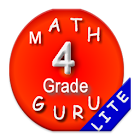All Android applications categories

All Android games categories152 6.6

6.6 Users
rating

## Screenshots

Description

Searching for math games for 4th graders ? Here it is. Fourth grade mathematics tests (Infinite numbers - System generated questions and answers,test, questions are Different every time) for 4th grade students.
This Fourth grade learning games contains syllabus based on Common Core math 4th grade math games free contains 4 Levels (Only one level in
Lite and all levels in Pro) to make your Kid Expert.

4.OA Word problems
• 4.OA Prime or composite
• 4.OA Patterns
• 4.NBT Place value and comparison
• 4.NBT Multiplication
• 4.NBT Division
• 4.NF Fractions
• 4.NF Fraction - addition - mixed numbers
• 4.NF Fraction - Subtraction
• 4.NF Fraction - subtraction - mixed numbers
• 4.NF Fraction - multiplication
• 4.NF Fractions - decimal notation
• 4.MD Measurement and data
• 4.G Geometry-1 , 2

CBSE Fourth grade maths common core syllabus - tests/ quiz/ worksheets of 4th grade math activities:
Worksheets / tests / quiz / 4th grade math activities covers common core syllabus 4.OA Operations and Algebraic Thinking
Interpret a multiplication equation as a comparison
free multiplication and division within 100 to solve word problems
four operations, and identify and explain patterns in arithmetic
Find all factor pairs -whole number - range 1–100

4th grade math common core syllabus 4.NBT Number and Operations in Base Ten with Worksheets / activities
place value for 4th graders to understand properties of operations to perform multi-digit arithmetic in baseten
add and subtract - place value, properties of operations, relationship between addition and subtraction in base ten
Multiply one-digit whole numbers by multiples of 10.
Compare two multi-digit numbers, round multi-digit whole numbers
Find whole-number quotients and remainders with up to four-digit dividends and one-digit divisors

Worksheets / tests / quiz / Fourth grade math activities - CBSE 4.NF Number and Operations Fractions
fractions as numbers for 4th grade students
Test understanding of fractions - fraction addition and fraction subtraction including word problems
fraction as a number on the number line
Express whole numbers as fraction
Compare two fractions-same & different numerator /denominator
multiply a fraction by a whole number
decimal notation for fractions, and compare decimal fractions.
Compare two decimals to hundredths

Worksheets - Fourth grade math activities- common core syllabus 4.MD Measurement and Data
conversion of measurements from a larger unit to a smaller unit
solve word problems involving distances, intervals of time, liquid volumes, masses of objects, and money
4th grade geometry : area of a rectangle,perimeter, Multiply side lengths
angles as geometric shapes that are formed wherever two rays share a common endpoint

Worksheets / tests / quiz / math games covers 4.G Geometry
points, lines, line segments, rays, angles (right, acute, obtuse), perpendicular, parallel lines, right triangles
Identify two-dimensional figures.
Solve problems related to reasoning with shapes and their attributes

This Fourth grade learning games free app, learn Mathematics wherever you are. 4th grade math testing preparation helps your kid to become expert.
This kids math games for Fourth grade is useful for school students of second grade, 4th grade and fourth grade to learn and test their understanding in one go.